## Year 3 Mental Arithmetic: Sets 61 and 62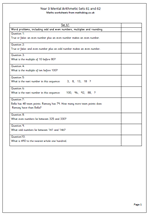This weeks’ mental arithmetic for year 3 has a variety of questions ranging from odd and even numbers to number sequences.

The Primary Framework for Mathematics lays great emphasis on odd and even numbers and it is very useful to know the pattern of adding and subtracting odd and even numbers, especially when carrying out quick checks as to whether answers are correct or not.

Children should know that:

an even number plus an even number will give and even number

an even number plus an odd number will give an odd number

an odd number plus an odd number will give an even number.

The same pattern occurs with subtraction.

The term ‘multiple’ is also expected to be understood in year 3 and in this early stage it can be thought of as:

‘a number produced by multiplying a starting whole number by another whole number’. The answers to times tables are excellent examples of multiples.

Year 3 mental arithmetic: Sets 61 and 62

## Year 4 maths worksheet: more on time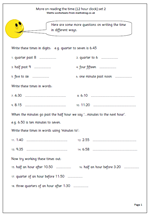By the end of year 4 it is expected that most children will be confident with reading the time but this is not always the case and this worksheet is a follow up to one published in April. It looks at reading the time using a 12 hour clock.

The first set of questions looks at writing times in digits, using the convention of separating the hours and minutes by a colon.

The second set of questions looks at writing times in words. Of course we can often do this in more than one way, and this can be confusing for children. For example: ‘5:50 can be said as ‘five fifty’ or ‘ten to six’. Interestingly we generally do not use minutes to the next hour for any time before the half hour. It sounds rather strange to say ’40 minutes to six’, although this is technically correct.

A favourite type of question in the SAT Papers is to ask what time it will be a quarter or half an hour before/after a given time. The last set of questions gives a little practice with this.

More on time (2)

## Year 3 mental artihmetic: sets 59 and 60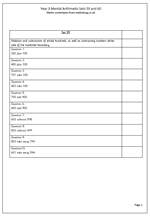As we move towards the end of the year 3 term the mental arithmetic questions become slightly harder. These two pages of addition and subtraction questions include some quite tricky problems. The first few questions are quite straightforward with adding or subtracting multiples of 100 from 3-digit numbers, although there are many children who are not at all comfortable with larger numbers. This can often be shown by asking them to count on in whole hundreds from a given number such as 34.

The last four questions look at subtraction either side of a multiple of 100. For example, at first glance 603 subtract 598 looks as if it should be done using paper and pencil methods, but it is quite easy to do by counting on.

Count on 2 from 598 to make 600. Then count on another 3 to make 603 which gives an answer of 5. No harm in using fingers to do this sort of question, especially if the question is given orally and the numbers cannot be seen.

Year 3 mental arithmetic_(sets 59 and 60)

## Counting on in steps of 10p, crossing the hundreds.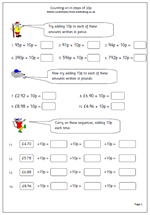I recently published a page on counting on in steps of 10p which was quite straightforward, but this one is much trickier. This time the counting on crosses the hundreds boundary and in the case of money this also crosses the decimal point.

The first set of questions just looks at 2-digit numbers which cross the hundreds boundary;

For example: 96p + 10p = 106p.

Questions 7 to 10 are much harder as they show the money as pounds rather than pence.

For example: £6.94 + 10p = £7.04.

There is quite a lot to remember here, including putting the pence as .04.

Some children will find this difficult and may well use their fingers to count on in single digits. Others will not convert to pounds properly; often answers such as this are seen:

£6.94 + 10p = £6.104

which shows a lack of understanding of the decimal system.

Count on in 10p crossing hundreds

## Maths board game: Four Stars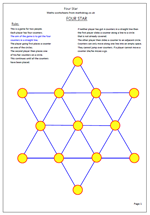I shall be publishing some fantastic strategy games over the next few weeks which can really help children develop their logical thinking and predicting what will happen. This is a great little game that does not need a lot of equipment and only takes a short time to play, but can get quite addictive. It’s a good idea to test out if going first or second leads to any advantage, and if so how this can be maximised. I will leave it up to you to decide!

Equipment:
Two sets of four counters.
A playing board
Rules:

This is a game for two people.
Each player has four counters.
The aim of the game is to get the four counters in a straight line.

The player going first places a counter on one of the circles.

Then the second player places one of his/her counters on a circle. This continues until all the counters have been placed.

If neither player has got 4 counters in a straight line then the first player slides a counter along a line to a circle that is not already covered.

The other player then slides a counter to an adjacent circle.  Counters can only move along one line into an empty space. They cannot jump over counters.

If a player cannot move a counter she/he misses a go.

This is best played at a fast pace and a time limit set for winning.

On the first page you will find a board which can be printed out onto card. It is a good idea to cut out and either laminate or ‘sticky back’ this board.

Four star

## Resource of the Week: Long division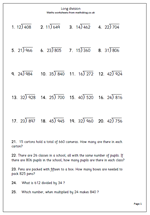If there is one thing which really proves to be tricky for primary school children it is long division. In fact there have been calls for it to be abandoned with the calculator taking over. However, if children do grasp the method they can get great satisfaction from working their way carefully through the method to reach a correct answer.

Long division is hard because it requires a good knowledge of tables,  the ability to multiply, estimate, use trial and improvement and go through several steps to reach the answer. These steps include:

e.g. 789 divided by 36

First carry out an estimate of the answer. I think 789 divided by 36 is about 20.

a. How many 36s in 78?

b. 2 x 36 is 72.    3 x 36 is 108 which is too many, so it must be 2.

c. Put the 2 in the tens column above the question.

d. Place the 72 below the 78 and subtract.

e. 78 – 72 is 6.

f. ‘Bring down’ the 9 to make 69.

g. How many 36s in 69.

h. By trial and improvement and some rough work multiplying 36 by my estimated numbers I find that 36 x 1 = 36.

i. Put the 1 in the units column above the question.

j. Place the 36 under the 69 and subtract.

k. The remainder (33) must be less than the original number you are dividing by.

I would suggest that only those children who have really mastered the basics of times tables and written methods for addition, subtraction and multiplication try these.

Division 3 by 2

## Year 3 Mental Arithmetic: Sets 57 and 58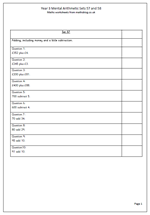We will soon be coming to the end of our sets of mental arithmetic questions for year 3, with just three more sets after these. Today the emphasis is on addition skills as well as a little subtraction.

The questions include:

adding a single digit to a 3-digit number in the context of money: e.g. £352 plus £4

adding a 2-digit number to a 3-digit multiple of 100: e.g. £400 + £88

adding a 2-digit multiple of 10 to a 2-digit number: e.g. to add 34

subtracting a single digit from a multiple of 100: e.g. 600 subtract 4

These are all important steps in becoming confident with working with numbers ‘in your head’ and they should be practiced as often as possible.

Year 3 mental arithmetic (sets 57 and 58)

## Resource of the Week: decimal fractions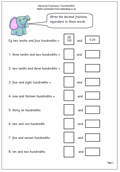Some children have difficulty realising that the same numbers can be written in different ways, especially when dealing with decimals and decimal fractions. This page is a good test of understanding, probably most suited for year 5 children (/10 yrs old).

0.23 can be read as 23 hundredths or 2 tenths and 3 hundredths. As a fraction it would be written as 23/100.

With a number such as four and three hundredths it is important to keep the zero in the tenths so that it is written as 4.03.

Watch out for a common mistake on a question such as thirteen hundredths when children write 0.013; the one digit has been placed in the hundredths column when it should be in the tenths with the 3 digit in the hundredths.

Decimal fractions: hundredths (pg 1)

## Maths worksheet: ordering amounts of money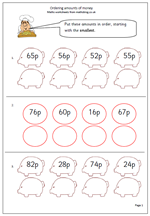Children in the Early Years get plenty of opportunity to handle coins and work on ordering small amounts of money. Yet, when children get older and the numbers get larger this is a concept which is often not practised in school. So here we have a maths worksheet which looks specifically at ordering 2-digit amounts of money written in pence.

There are four amounts in each row and it is best to take a step by step approach; first of all finding the smallest number, writing it in the answer column and then crossing it out on the top row. Crossing out is important as it can easily be seen that that number has been eliminated. Then proceed to the next number and so on.

Order money

## Year 3 mental arithmetic: sets 55 and 56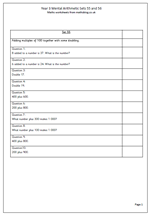Two more sets of ten questions which add to the great collection now available for year 3 children. This week there are some tricky questions which must be listened to carefully if the correct answer is to be worked out.

For example: ‘8 added to a number is 37. What is the number?’

The danger here is to add 8 to 37 rather than subtract it!

other questions cover doubling numbers in the teens. This is quite hard for year 3 children but it is hoped that they learn the answers to these off by heart as soon as possible. The rest of the set are mainly concerned with the addition of multiples of 100.

Year 3_mental arithmetic:_(sets 55 and 56)